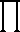# Math2.org Math Tables:(Math)

 An Introduction to  Generalized Calculus Unit I - Product Calculus Section B - Discrete Product

# I. Product Calculus

## B. Discrete Product

The discrete product, as I will name it for classification purposes, is widely used already, though not classified as a component of product calculus, so I will only review it. The next section builds heavily upon the discrete product.

The discrete product,, of a function f(x) is simply defined as:f(k) = f(1) f(2) f(3) ... f(x)
x

simularly, we can place a lower limit a on the product:

xf(k) = f(a) f(a+1) f(a+2) ... f(x)
k=a

A product we deal with often is x!, written in product notation as:k = (1)(2)(3) ... (x) = x!
x

or alternately written,

xk = (1)(2)(3) ... (x) = x!
k=1

Next, we must convert this discrete product into its continuous form.